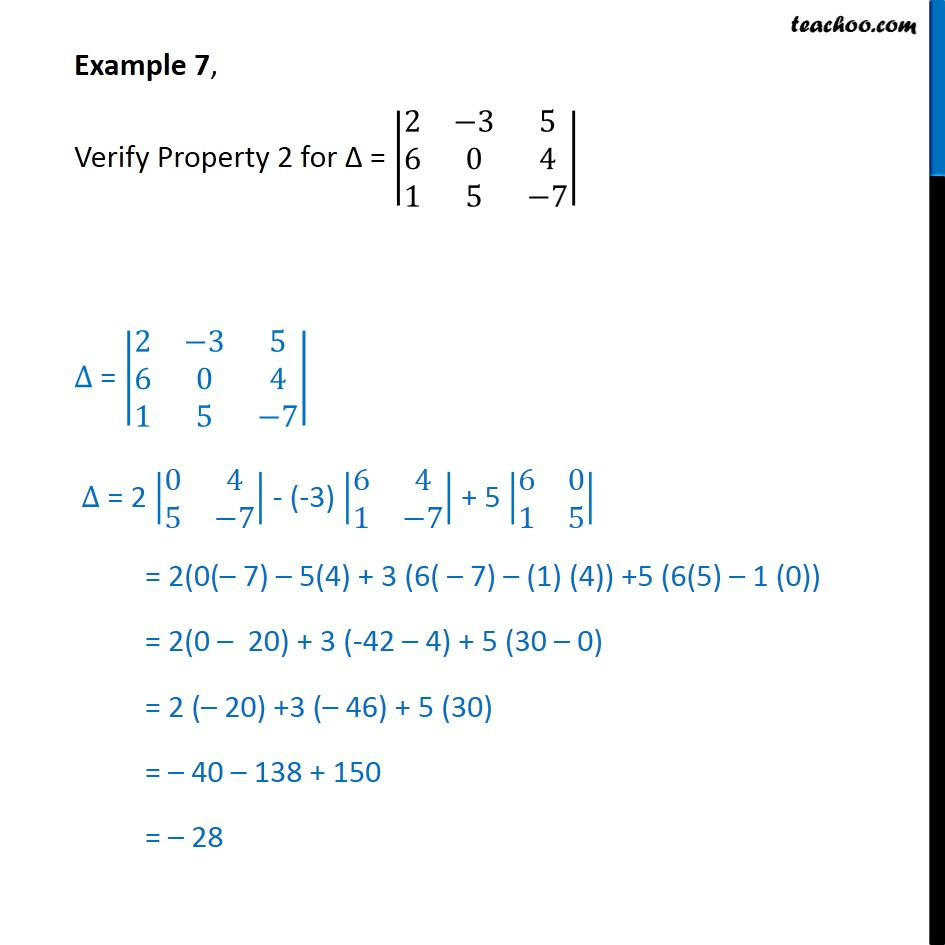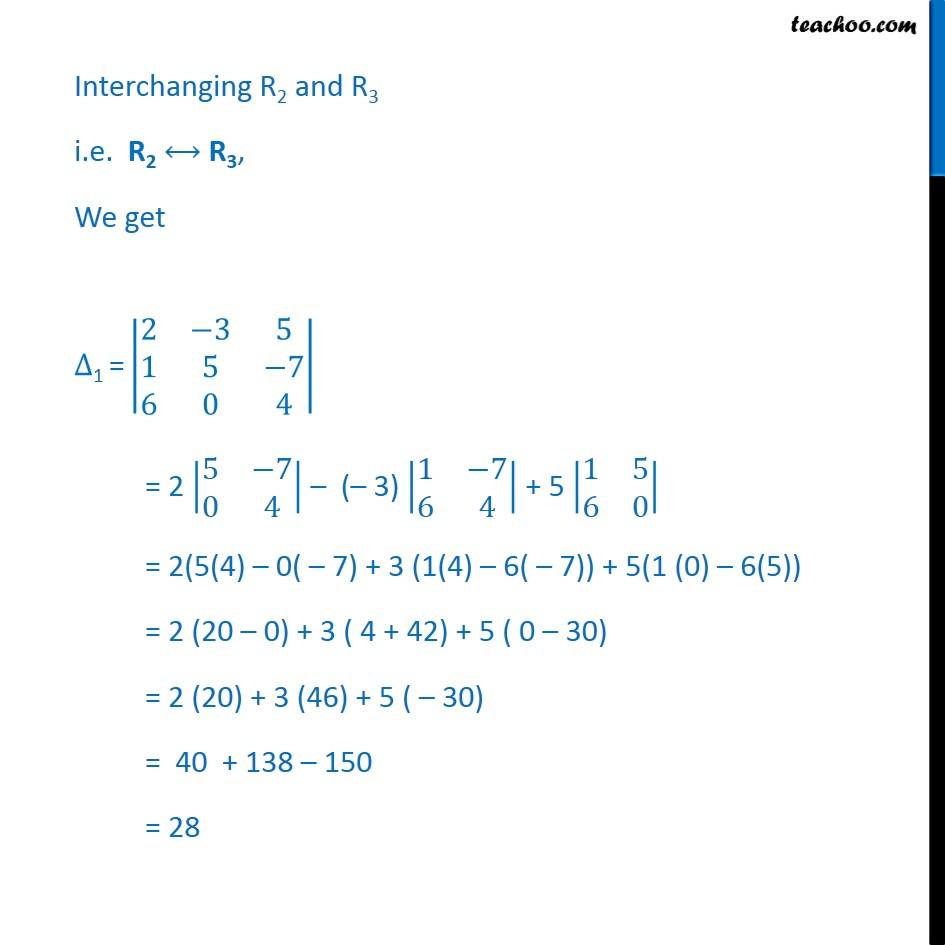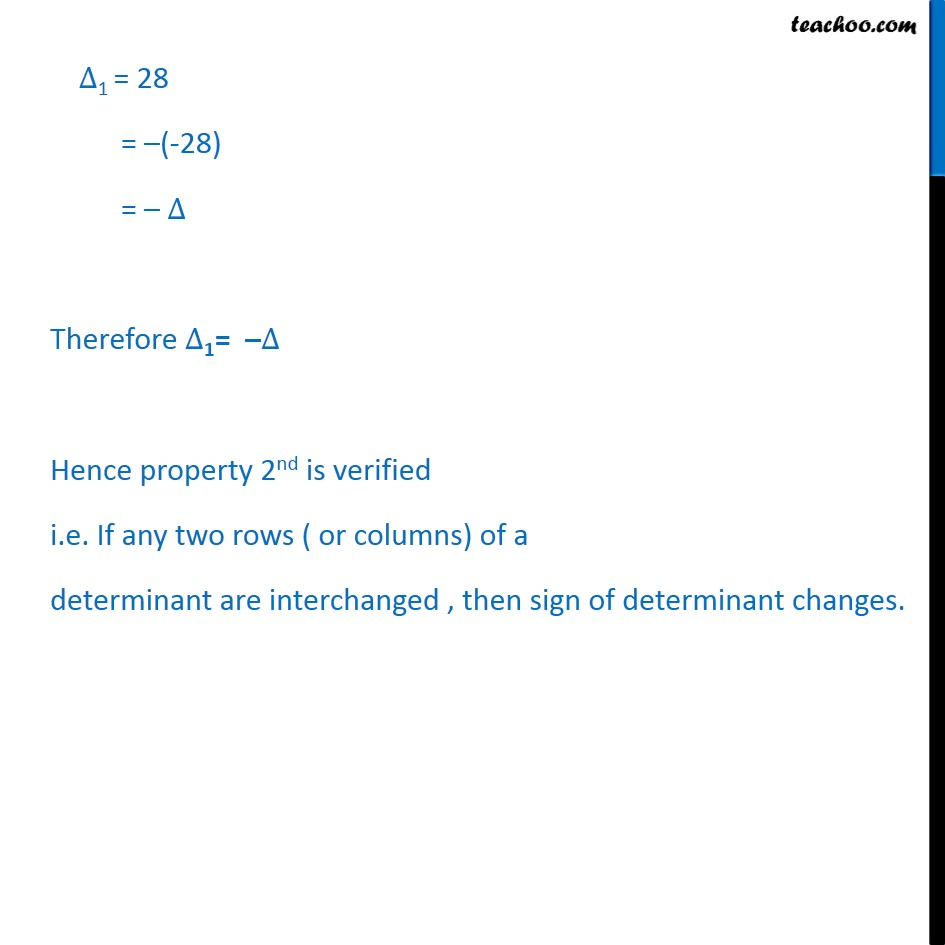Verifying properties of a determinant

Chapter 4 Class 12 Determinants
Concept wiseLearn in your speed, with individual attention - Teachoo Maths 1-on-1 Class

### Transcript

Question 2, Verify Property 2 for = 2 3 5 6 0 4 1 5 7 = 2 3 5 6 0 4 1 5 7 = 2 0 4 5 7 - (-3) 6 4 1 7 + 5 6 0 1 5 = 2(0( 7) 5(4) + 3 (6( 7) (1) (4)) +5 (6(5) 1 (0)) = 2(0 20) + 3 (-42 4) + 5 (30 0) = 2 ( 20) +3 ( 46) + 5 (30) = 40 138 + 150 = 28 Interchanging R2 and R3 i.e. R2 R3, We get 1 = 2 3 5 1 5 7 6 0 4 = 2 5 7 0 4 ( 3) 1 7 6 4 + 5 1 5 6 0 = 2(5(4) 0( 7) + 3 (1(4) 6( 7)) + 5(1 (0) 6(5)) = 2 (20 0) + 3 ( 4 + 42) + 5 ( 0 30) = 2 (20) + 3 (46) + 5 ( 30) = 40 + 138 150 = 28 1 = 28 = (-28) = Therefore 1= Hence property 2nd is verified i.e. If any two rows ( or columns) of a determinant are interchanged , then sign of determinant changes.